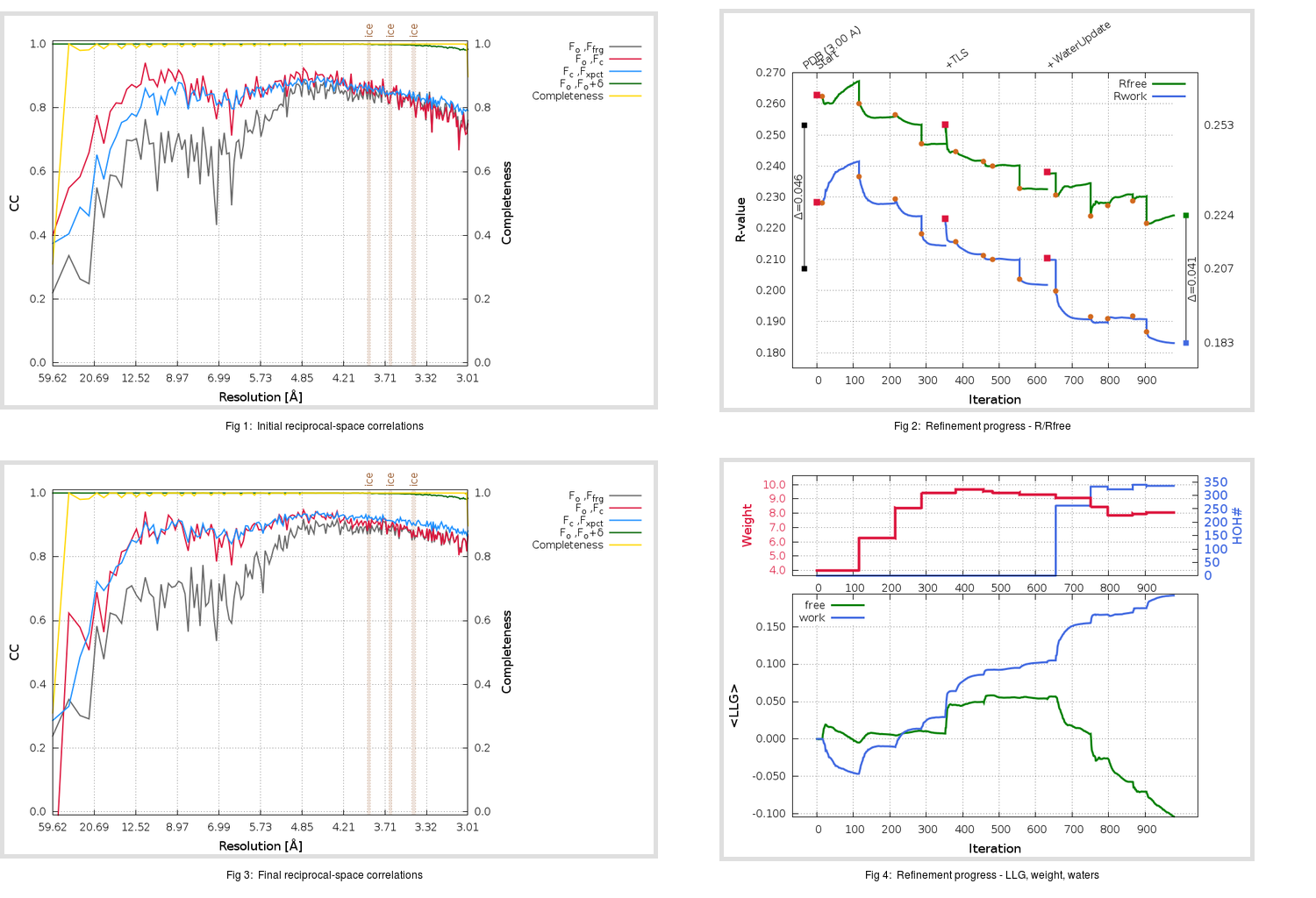Content:

```    Diffraction limits & principal axes of ellipsoid fitted to diffraction cut-off surface:
2.959         1.0000   0.0000   0.0000       0.894 a* - 0.447 b*
2.959         0.0000   1.0000   0.0000       b*
2.982         0.0000   0.0000   1.0000       c*
```

## Deposited

` `
 Date deposited Date data collection Resolution R, Rfree 20130613 20130405 3.00 0.2050 0.2530

Molprobity (CCP4 7.0 version) summary:

```Ramachandran outliers =   0.86 %
favored =  92.13 %
Rotamer outliers      =   8.70 %
C-beta deviations     =     4
Clashscore            =   6.64
RMS(bonds)            =   0.0097
RMS(angles)           =   1.36
MolProbity score      =   2.56
Resolution            =   3.00
R-work                =   0.2050
R-free                =   0.2530
```

```Number of waters      =     0

<B> (all atoms) =   24.60 ( sd =   14.60 ) for       7705 non-hydrogen atoms
<B>   (protein) =   24.80 ( sd =   14.70 ) for       7540 non-hydrogen atoms
<B>     (water) =    0.00 ( sd =    0.00 ) for          0 non-hydrogen atoms
<B>    (others) =   15.80 ( sd =    0.00 ) for        165 non-hydrogen atoms

B min/max       (all non-hydrogen atoms) =    6.76 /   84.47
B min/max   (protein non-hydrogen atoms) =    6.76 /   84.47
B min/max     (water non-hydrogen atoms) =    0.00 /    0.00
B min/max     (other non-hydrogen atoms) =   15.80 /   15.80
```

## BUSTER (re-)refinement

` `

Molprobity (CCP4 7.0 version) summary:

```Ramachandran outliers =   0.54 %
favored =  94.18 %
Rotamer outliers      =   7.27 %
C-beta deviations     =     7
Clashscore            =   4.91
RMS(bonds)            =   0.0123
RMS(angles)           =   1.69
MolProbity score      =   2.30
Resolution            =   3.00
R-work                =   0.1831
R-free                =   0.2241
```

```Number of waters      =   336

<B> (all atoms) =   47.22 ( sd =   18.16 ) for       8041 non-hydrogen atoms
<B>   (protein) =   47.12 ( sd =   18.25 ) for       7540 non-hydrogen atoms
<B>     (water) =   41.90 ( sd =   13.78 ) for        336 non-hydrogen atoms
<B>    (others) =   62.24 ( sd =   13.07 ) for        165 non-hydrogen atoms

B min/max       (all non-hydrogen atoms) =    8.34 /  125.37
B min/max   (protein non-hydrogen atoms) =   19.76 /  125.37
B min/max     (water non-hydrogen atoms) =    8.34 /  125.07
B min/max     (other non-hydrogen atoms) =   35.65 /   87.61
```

Refinement progression:Results:

` `
 File Remark 4L72_aB_refine.01_03_refine.pdb.gz exact refinement commands are in header 4L72_aB_refine.01_03_refine.mtz.gz including original deposited data and several re-refinement map coefficients 4L72_aB_refine.01_03_BUSTER_model.cif.gz including any non-standard compound restraints 4L72_aB_refine.01_03_BUSTER_refln.cif.gz